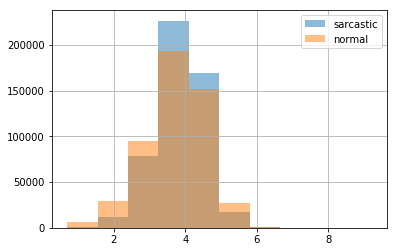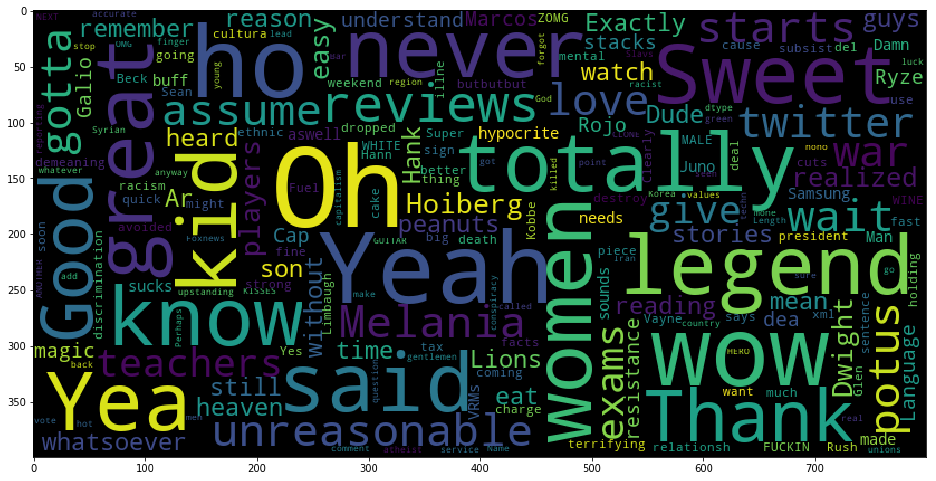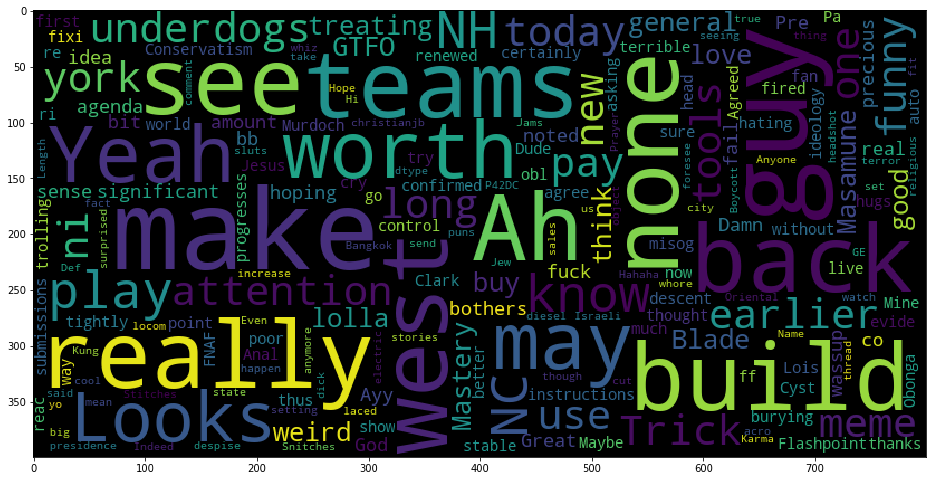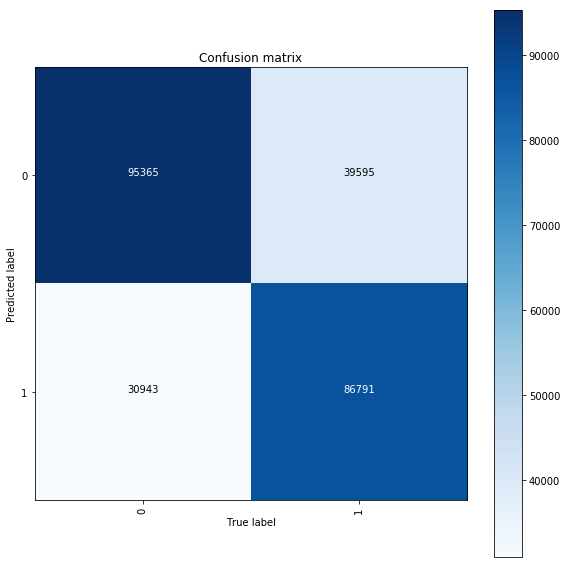## Assignment 4 (demo). Solution

### Sarcasm detection with logistic regression

Same assignment as a Kaggle Kernel + solution.

We'll be using the dataset from the paper "A Large Self-Annotated Corpus for Sarcasm" with >1mln comments from Reddit, labeled as either sarcastic or not. A processed version can be found on Kaggle in a form of a Kaggle Dataset.

In :
PATH_TO_DATA = '../input/sarcasm/train-balanced-sarcasm.csv'

In :
# some necessary imports
import os
import numpy as np
import pandas as pd
from sklearn.feature_extraction.text import TfidfVectorizer
from sklearn.linear_model import LogisticRegression
from sklearn.pipeline import Pipeline
from sklearn.model_selection import train_test_split
from sklearn.metrics import accuracy_score, confusion_matrix
import seaborn as sns
from matplotlib import pyplot as plt

In :
train_df = pd.read_csv(PATH_TO_DATA)

In :
train_df.head()

Out:
label comment author subreddit score ups downs date created_utc parent_comment
0 0 NC and NH. Trumpbart politics 2 -1 -1 2016-10 2016-10-16 23:55:23 Yeah, I get that argument. At this point, I'd ...
1 0 You do know west teams play against west teams... Shbshb906 nba -4 -1 -1 2016-11 2016-11-01 00:24:10 The blazers and Mavericks (The wests 5 and 6 s...
2 0 They were underdogs earlier today, but since G... Creepeth nfl 3 3 0 2016-09 2016-09-22 21:45:37 They're favored to win.
3 0 This meme isn't funny none of the "new york ni... icebrotha BlackPeopleTwitter -8 -1 -1 2016-10 2016-10-18 21:03:47 deadass don't kill my buzz
4 0 I could use one of those tools. cush2push MaddenUltimateTeam 6 -1 -1 2016-12 2016-12-30 17:00:13 Yep can confirm I saw the tool they use for th...
In :
train_df.info()

<class 'pandas.core.frame.DataFrame'>
RangeIndex: 1010826 entries, 0 to 1010825
Data columns (total 10 columns):
label             1010826 non-null int64
comment           1010773 non-null object
author            1010826 non-null object
subreddit         1010826 non-null object
score             1010826 non-null int64
ups               1010826 non-null int64
downs             1010826 non-null int64
date              1010826 non-null object
created_utc       1010826 non-null object
parent_comment    1010826 non-null object
dtypes: int64(4), object(6)
memory usage: 77.1+ MB


Some comments are missing, so we drop the corresponding rows.

In :
train_df.dropna(subset=['comment'], inplace=True)


We notice that the dataset is indeed balanced

In :
train_df['label'].value_counts()

Out:
0    505405
1    505368
Name: label, dtype: int64

We split data into training and validation parts.

In :
train_texts, valid_texts, y_train, y_valid = \
train_test_split(train_df['comment'], train_df['label'], random_state=17)


1. Analyze the dataset, make some plots. This Kernel might serve as an example
2. Build a Tf-Idf + logistic regression pipeline to predict sarcasm (label) based on the text of a comment on Reddit (comment).
3. Plot the words/bigrams which a most predictive of sarcasm (you can use eli5 for that)
4. (optionally) add subreddits as new features to improve model performance. Apply here the Bag of Words approach, i.e. treat each subreddit as a new feature.

### Part 1. Exploratory data analysis¶

Distribution of lengths for sarcastic and normal comments is almost the same.

In :
train_df.loc[train_df['label'] == 1, 'comment'].str.len().apply(np.log1p).hist(label='sarcastic', alpha=.5)
train_df.loc[train_df['label'] == 0, 'comment'].str.len().apply(np.log1p).hist(label='normal', alpha=.5)
plt.legend();In :
from wordcloud import WordCloud, STOPWORDS

In :
wordcloud = WordCloud(background_color='black', stopwords = STOPWORDS,
max_words = 200, max_font_size = 100,
random_state = 17, width=800, height=400)


Word cloud are nice, but not very useful

In :
plt.figure(figsize=(16, 12))
wordcloud.generate(str(train_df.loc[train_df['label'] == 1, 'comment']))
plt.imshow(wordcloud);In :
plt.figure(figsize=(16, 12))
wordcloud.generate(str(train_df.loc[train_df['label'] == 0, 'comment']))
plt.imshow(wordcloud);Let's analyze whether some subreddits are more "sarcastic" on average than others

In :
sub_df = train_df.groupby('subreddit')['label'].agg([np.size, np.mean, np.sum])

Out:
size mean sum
subreddit
politics 39493 0.605348 23907
worldnews 26376 0.642516 16947
leagueoflegends 21034 0.542312 11407
pcmasterrace 18987 0.566651 10759
news 16891 0.603457 10193
funny 17939 0.451474 8099
pics 16152 0.484336 7823
todayilearned 14159 0.547567 7753
GlobalOffensive 13738 0.552045 7584
In :
sub_df[sub_df['size'] > 1000].sort_values(by='mean', ascending=False).head(10)

Out:
size mean sum
subreddit
creepyPMs 5466 0.784303 4287
MensRights 3355 0.680775 2284
ShitRedditSays 1284 0.661994 850
worldnews 26376 0.642516 16947
Libertarian 2562 0.640125 1640
atheism 7377 0.639555 4718
Conservative 1881 0.639553 1203
TwoXChromosomes 1560 0.632692 987
fatlogic 2356 0.623090 1468
facepalm 1268 0.617508 783

The same for authors doesn't yield much insight. Except for the fact that somebody's comments were sampled - we can see the same amounts of sarcastic and non-sarcastic comments.

In :
sub_df = train_df.groupby('author')['label'].agg([np.size, np.mean, np.sum])

Out:
size mean sum
author
NeonDisease 422 0.500000 211
ShyBiDude89 404 0.500000 202
ivsciguy 342 0.500000 171
mindlessrabble 302 0.500000 151
pokemon_fetish 432 0.500000 216
Biffingston 845 0.499408 422
In :
sub_df = train_df[train_df['score'] >= 0].groupby('score')['label'].agg([np.size, np.mean, np.sum])

Out:
size mean sum
score
0 58141 0.612752 35626
20 3096 0.561370 1738
34 1071 0.556489 596
26 1874 0.554429 1039
59 315 0.552381 174
18 3951 0.551759 2180
13 7277 0.546929 3980
17 4404 0.545867 2404
40 722 0.545706 394
27 1753 0.545351 956
In :
sub_df = train_df[train_df['score'] < 0].groupby('score')['label'].agg([np.size, np.mean, np.sum])

Out:
size mean sum
score
-14 537 0.698324 375
-8 1852 0.696004 1289
-9 1503 0.691284 1039
-6 3088 0.688795 2127
-12 776 0.684278 531
-5 4134 0.678520 2805
-3 6736 0.676069 4554
-2 11924 0.675948 8060
-4 5436 0.673473 3661
-7 2424 0.672855 1631

### Part 2. Training the model¶

In :
# build bigrams, put a limit on maximal number of features
# and minimal word frequency
tf_idf = TfidfVectorizer(ngram_range=(1, 2), max_features=50000, min_df=2)
# multinomial logistic regression a.k.a softmax classifier
logit = LogisticRegression(C=1, n_jobs=4, solver='lbfgs',
random_state=17, verbose=1)
# sklearn's pipeline
tfidf_logit_pipeline = Pipeline([('tf_idf', tf_idf),
('logit', logit)])

In :
%%time
tfidf_logit_pipeline.fit(train_texts, y_train)

[Parallel(n_jobs=4)]: Using backend LokyBackend with 4 concurrent workers.

CPU times: user 39.6 s, sys: 2.73 s, total: 42.3 s
Wall time: 1min 7s

[Parallel(n_jobs=4)]: Done   1 out of   1 | elapsed:   26.1s finished

Out:
Pipeline(memory=None,
steps=[('tf_idf', TfidfVectorizer(analyzer='word', binary=False, decode_error='strict',
dtype=<class 'numpy.float64'>, encoding='utf-8', input='content',
lowercase=True, max_df=1.0, max_features=50000, min_df=2,
ngram_range=(1, 2), norm='l2', preprocessor=None, smooth_idf=Tru... penalty='l2', random_state=17, solver='lbfgs', tol=0.0001,
verbose=1, warm_start=False))])
In :
%%time
valid_pred = tfidf_logit_pipeline.predict(valid_texts)

CPU times: user 6.53 s, sys: 80 ms, total: 6.61 s
Wall time: 6.6 s

In :
accuracy_score(y_valid, valid_pred)

Out:
0.7208560551497067

### Part 3. Explaining the model¶

In :
def plot_confusion_matrix(actual, predicted, classes,
normalize=False,
title='Confusion matrix', figsize=(7,7),
cmap=plt.cm.Blues, path_to_save_fig=None):
"""
This function prints and plots the confusion matrix.
Normalization can be applied by setting normalize=True.
"""
import itertools
from sklearn.metrics import confusion_matrix
cm = confusion_matrix(actual, predicted).T
if normalize:
cm = cm.astype('float') / cm.sum(axis=1)[:, np.newaxis]

plt.figure(figsize=figsize)
plt.imshow(cm, interpolation='nearest', cmap=cmap)
plt.title(title)
plt.colorbar()
tick_marks = np.arange(len(classes))
plt.xticks(tick_marks, classes, rotation=90)
plt.yticks(tick_marks, classes)

fmt = '.2f' if normalize else 'd'
thresh = cm.max() / 2.
for i, j in itertools.product(range(cm.shape), range(cm.shape)):
plt.text(j, i, format(cm[i, j], fmt),
horizontalalignment="center",
color="white" if cm[i, j] > thresh else "black")

plt.tight_layout()
plt.ylabel('Predicted label')
plt.xlabel('True label')

if path_to_save_fig:
plt.savefig(path_to_save_fig, dpi=300, bbox_inches='tight')


Confusion matrix is quite balanced.

In :
plot_confusion_matrix(y_valid, valid_pred,
tfidf_logit_pipeline.named_steps['logit'].classes_, figsize=(8, 8))Indeed, we can recognize some phrases indicative of sarcasm. Like "yes sure".

In :
import eli5
eli5.show_weights(estimator=tfidf_logit_pipeline.named_steps['logit'],
vec=tfidf_logit_pipeline.named_steps['tf_idf'])

Out:

y=1 top features

Weight? Feature
+9.328 yes because
+7.904 clearly
+7.374 obviously
+7.268 yeah because
+6.880 totally
+6.192 because
+6.080 how dare
+5.935 right because
+5.405 but thought
+5.234 duh
+4.915 good thing
+4.797 gee
+4.752 yes let
+4.627 therefore
+4.600 everyone knows
+4.567 fault
… 27225 more positive …
… 22756 more negative …
-4.942 fair enough
-5.032 iirc
-5.039 true but

So sarcasm detection is easy.### Part 4. Improving the model¶

In :
subreddits = train_df['subreddit']
train_subreddits, valid_subreddits = train_test_split(subreddits, random_state=17)


We'll have separate Tf-Idf vectorizers for comments and for subreddits. It's possible to stick to a pipeline as well, but in that case it becomes a bit less straightforward. Example

In :
tf_idf_texts = TfidfVectorizer(ngram_range=(1, 2), max_features=50000, min_df=2)
tf_idf_subreddits = TfidfVectorizer(ngram_range=(1, 1))


Do transformations separately for comments and subreddits.

In :
%%time
X_train_texts = tf_idf_texts.fit_transform(train_texts)
X_valid_texts = tf_idf_texts.transform(valid_texts)

CPU times: user 45.7 s, sys: 2.12 s, total: 47.8 s
Wall time: 47.8 s

In :
X_train_texts.shape, X_valid_texts.shape

Out:
((758079, 50000), (252694, 50000))
In :
%%time
X_train_subreddits = tf_idf_subreddits.fit_transform(train_subreddits)
X_valid_subreddits = tf_idf_subreddits.transform(valid_subreddits)

CPU times: user 3.48 s, sys: 0 ns, total: 3.48 s
Wall time: 3.48 s

In :
X_train_subreddits.shape, X_valid_subreddits.shape

Out:
((758079, 13255), (252694, 13255))

Then, stack all features together.

In :
from scipy.sparse import hstack
X_train = hstack([X_train_texts, X_train_subreddits])
X_valid = hstack([X_valid_texts, X_valid_subreddits])

In :
X_train.shape, X_valid.shape

Out:
((758079, 63255), (252694, 63255))

Train the same logistic regression.

In :
logit.fit(X_train, y_train)

[Parallel(n_jobs=4)]: Using backend LokyBackend with 4 concurrent workers.
[Parallel(n_jobs=4)]: Done   1 out of   1 | elapsed:   22.8s finished

Out:
LogisticRegression(C=1, class_weight=None, dual=False, fit_intercept=True,
intercept_scaling=1, max_iter=100, multi_class='warn', n_jobs=4,
penalty='l2', random_state=17, solver='lbfgs', tol=0.0001,
verbose=1, warm_start=False)
In :
%%time
valid_pred = logit.predict(X_valid)

CPU times: user 168 ms, sys: 0 ns, total: 168 ms
Wall time: 167 ms

In :
accuracy_score(y_valid, valid_pred)

Out:
0.7237647114692078

As we can see, accuracy slightly increased.